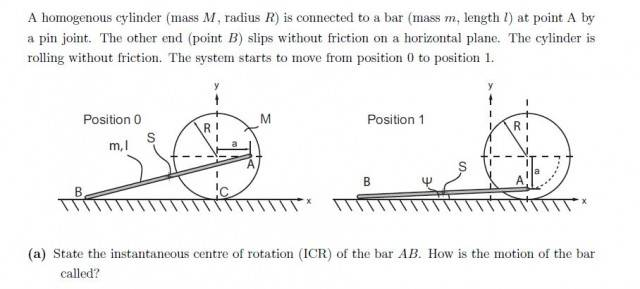# Instant centre of rotation

• Hidd

## Homework Statement## Homework Equations

orthogonality condition: that means that the point of ICR is orthogonal to the velocity Vb and Va

## The Attempt at a Solution

the solution that i found with the problem is:
The ICR of the bar is at infinity. the motion of the bar is translational.

I think that the answer is wrong because the point A is rotating, thus the the velocity of vb is not parallel to Va.

#### Attachments

•